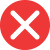20th

Correct spelling, explanation: ordinal numbers are usually created by adding “th” to the end of the cardinal number. Sometimes other letters in the number name are changed due to pronunciation, but generally adding this suffix is the universal rule. The exceptions are 1, 2 and 3 (ordinal numbers are: 1st – first, 2nd – second, 3rd – third), which end with st, nd and rd also when are the ending of a bigger number (for example 22nd, 43rd, 191st). Number 20 doesn’t end with any of these, so the suffix for the ordinal form is -th. Therefore 20th is the correct form, and 20nd doesn’t exist.

Definition of 20th:
numeral, ordinal number between 19th and 21st
My aunt is crazy about cats – this one is her 20th.
I want to fly a balloon by my 20th birthday.20nd

Incorrect spelling, explanation: 20nd is a wrong form, because cardinal number 20 doesn’t end with 2, so the ordinal number suffix is -th, as for the most numbers. The correct form, thus, is 20th. If the number ends with 1, 2 or 3, the endings are respectively -st, -nd and -rd. Suffix for any other ordinal number, not ending with these three, is -th.20rd

Incorrect spelling20st

Incorrect spelling